Next: 2.3 Constitutive Relation Formulation Up: 2. Circuit Equations Previous: 2.1 Nodal Approach

# 2.2 Modified Nodal Approach

The modified nodal approach (MNA)  overcomes the above stated shortcomings by introducing branch currents as independent variables which are available to formulate the device constitutive relations. The MNA enjoyed large popularity due to its simplicity and ease of implementation and is employed in SPICE which can safely be labeled the standard simulation program. However, the numerically well behaved circuit matrix obtained by the NA is distorted by those additional equations, and some additional measures have to be taken to allow for the use of iterative solvers.

An ideal voltage source introduces a direct relationship between the node voltages it is connected to of the form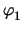-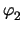= V0   . (2.16)

The current flowing through this source is added as an additional unknown and is used to formulated KCL at the respective nodes. Hence, the following stamp is obtained

 yx, yI r n1 -1 n2 1 I -1 1 V0
As pointed out above, these additional equations can produce a zero entry in the main-diagonal, hence an iterative solver cannot be used directly. Zero diagonals can be avoided by exchanging the rows of the admittance matrix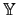following a simple algorithm  which guarantees non-zero main-diagonal entries. Failure of this algorithm denotes voltage source loops which indicate an error in the circuit description.

In general, the MNA matrix can be expressed in the form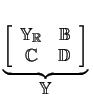.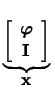=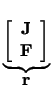(2.17)

where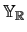is a reduced form of the nodal matrix excluding the contributions due to voltage sources, current controlling elements, etc.,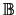contains partial derivatives of the Kirchhoff current equations with respect to the additional current variables which are normally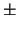1. The additional branch constitutive relations, derived with respect to the unknown vector are represented by the matricesand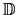. The vectors J and F are the excitation vectors. From an implementations point of view it is advantageous to consider the contributions of each circuit element to the MNA matrix separately.Next: 2.3 Constitutive Relation Formulation Up: 2. Circuit Equations Previous: 2.1 Nodal Approach
Tibor Grasser
1999-05-31